# The DD OO Recipe CS 5010 Program Design

• Slides: 21Introduction • We’ve seen the connections: – objects are like structs – classes are like define-structs – interfaces are like data definitions • We’ve seen that an OO program is just like a functional program, but with the pieces arranged in a different way • If I had a functional program, just what would I need to do to convert it to an OO program? 2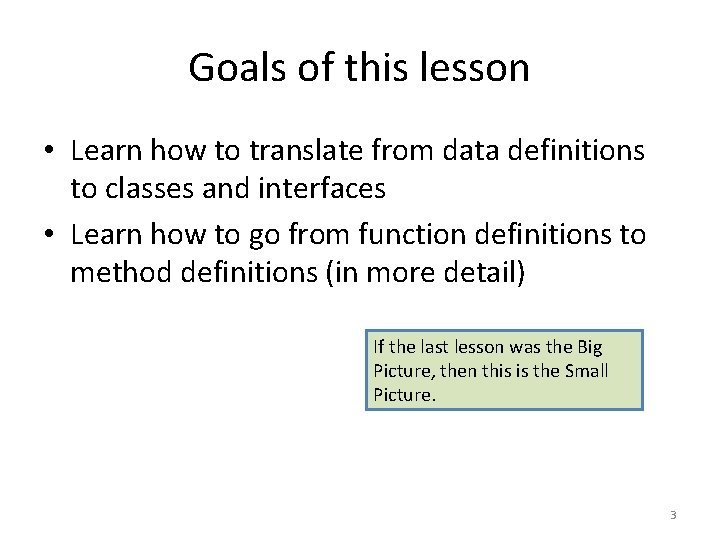Goals of this lesson • Learn how to translate from data definitions to classes and interfaces • Learn how to go from function definitions to method definitions (in more detail) If the last lesson was the Big Picture, then this is the Small Picture. 3Objects and Classes as Data Designs • Objects and Classes provide a new representation for information. • This representation is different from the one we have been using, but the correspondence between the two representations is simple. • Here it is: 4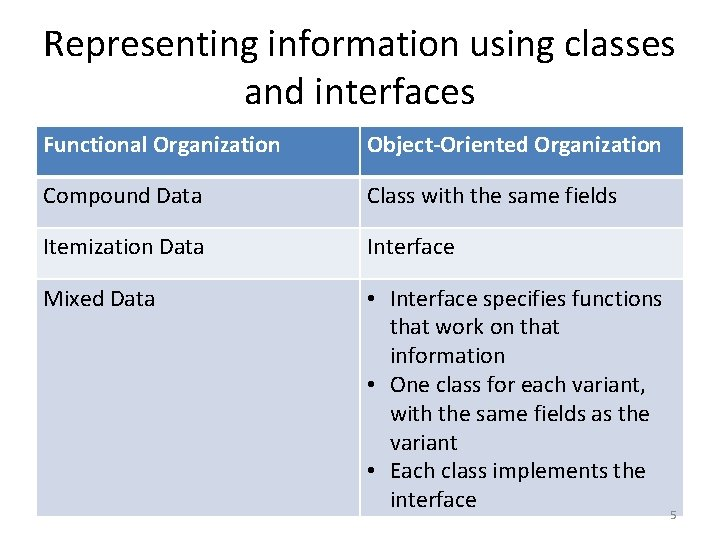Representing information using classes and interfaces Functional Organization Object-Oriented Organization Compound Data Class with the same fields Itemization Data Interface Mixed Data • Interface specifies functions that work on that information • One class for each variant, with the same fields as the variant • Each class implements the interface 5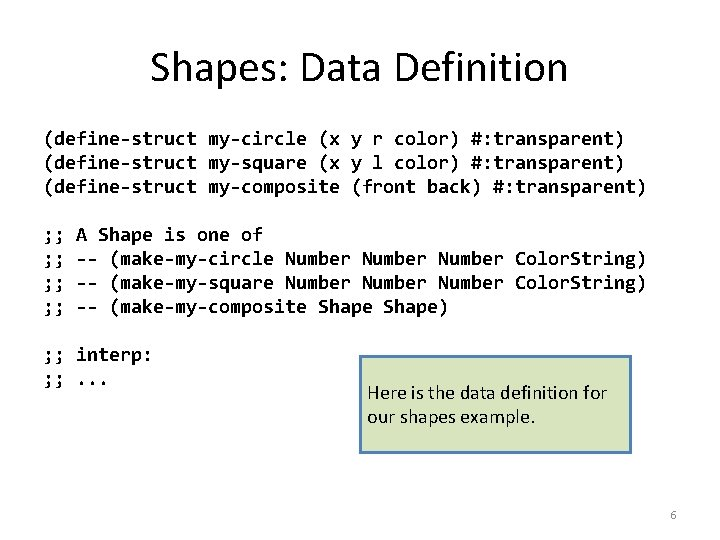Shapes: Data Definition (define-struct my-circle (x y r color) #: transparent) (define-struct my-square (x y l color) #: transparent) (define-struct my-composite (front back) #: transparent) ; ; ; ; A Shape is one of -- (make-my-circle Number Color. String) -- (make-my-square Number Color. String) -- (make-my-composite Shape) ; ; interp: ; ; . . . Here is the data definition for our shapes example. 6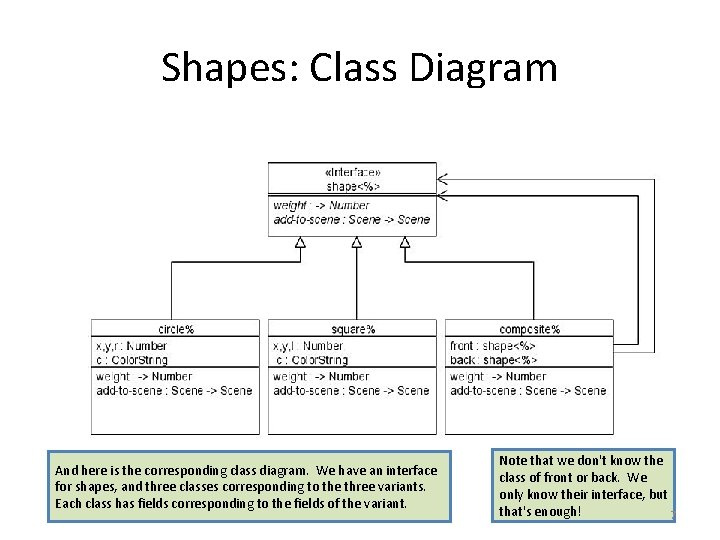Shapes: Class Diagram And here is the corresponding class diagram. We have an interface for shapes, and three classes corresponding to the three variants. Each class has fields corresponding to the fields of the variant. Note that we don't know the class of front or back. We only know their interface, but that's enough! 7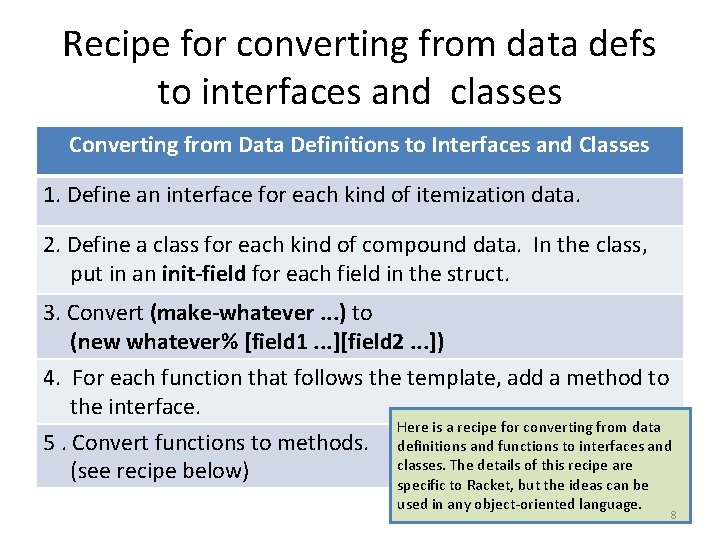Recipe for converting from data defs to interfaces and classes Converting from Data Definitions to Interfaces and Classes 1. Define an interface for each kind of itemization data. 2. Define a class for each kind of compound data. In the class, put in an init-field for each field in the struct. 3. Convert (make-whatever. . . ) to (new whatever% [field 1. . . ][field 2. . . ]) 4. For each function that follows the template, add a method to the interface. Here is a recipe for converting from data 5. Convert functions to methods. definitions and functions to interfaces and classes. The details of this recipe are (see recipe below) specific to Racket, but the ideas can be used in any object-oriented language. 8Example (define-struct foo (field 1 left right)) (define-struct bar (lo hi)) ; ; Data Definition ; ; A Baz is one of ; ; -- (make-foo Number Baz) ; ; -- (make-bar Number) Here’s an example of steps 1 and 2 of the recipe. The compound data Baz, with variants foo and bar, turns into an interface Baz<%> , with classes Foo% and Bar% that Implement Baz<%>. (define Baz<%> (interface (). . . )) (define Foo% (class* object% (Baz<%>) (init-field 1 left right). . . (super-new))) (define Bar% (class* object% (Baz<%>) (init-field lo hi). . . 9 (super-new)))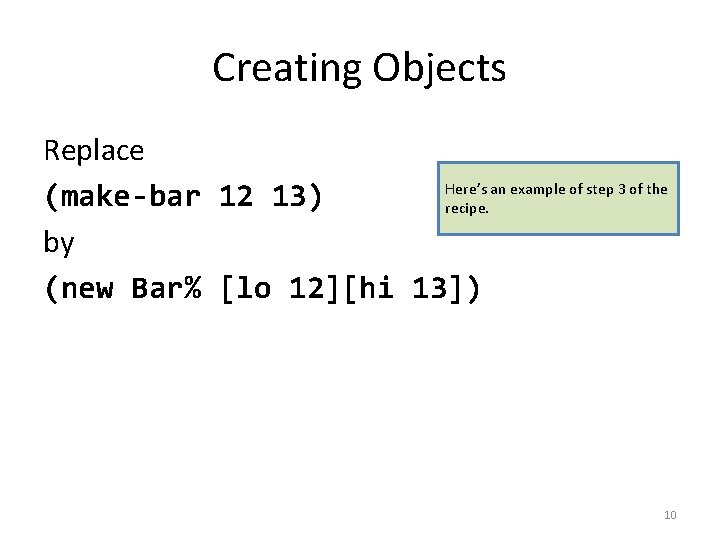Creating Objects Replace Here’s an example of step 3 of the (make-bar 12 13) recipe. by (new Bar% [lo 12][hi 13]) 10From function calls to method calls Instead of saying (baz-fn a-baz n) say (send a-baz fn n) Think: "Send John a book" (not "send a book to John"). 11From Function Definitions to Method Definitions Converting a Function Definition to a Method Definition 1. Add function name to interface 2. Pull out the parts 3. Change selectors to field references 4. Change function calls to method calls 5. Put method definitions into classes Here is a recipe for converting a function definition to a method definition. This expands step 5 of the recipe above. 12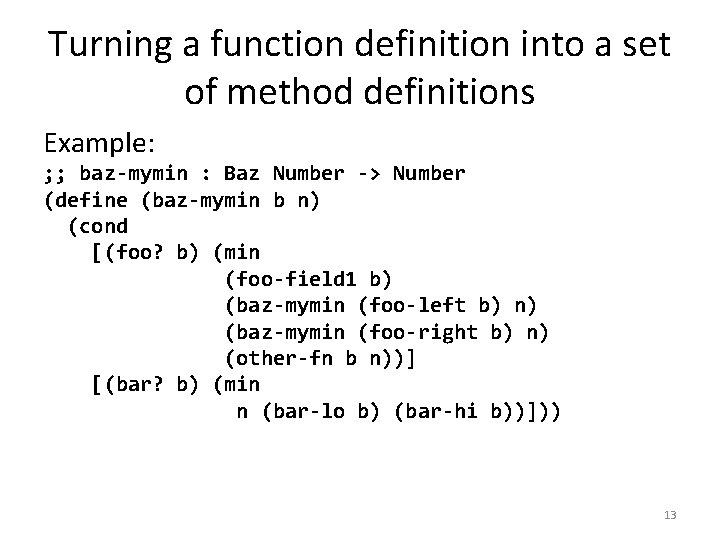Turning a function definition into a set of method definitions Example: ; ; baz-mymin : Baz Number -> Number (define (baz-mymin b n) (cond [(foo? b) (min (foo-field 1 b) (baz-mymin (foo-left b) n) (baz-mymin (foo-right b) n) (other-fn b n))] [(bar? b) (min n (bar-lo b) (bar-hi b))])) 13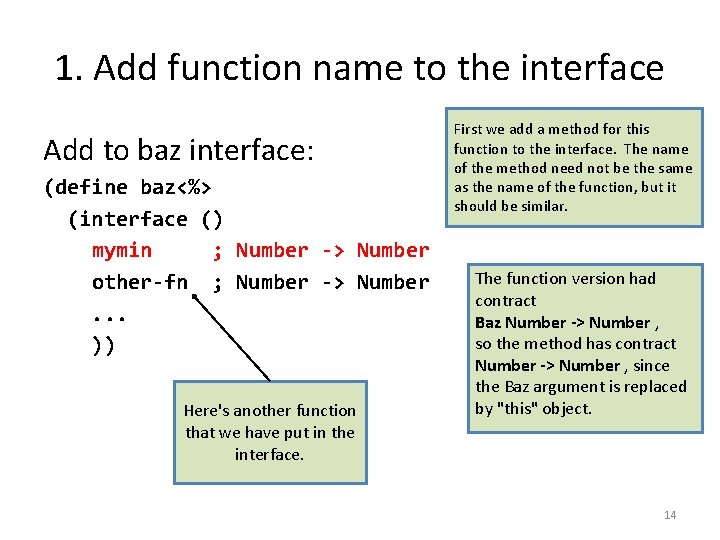1. Add function name to the interface Add to baz interface: (define baz<%> (interface () mymin ; Number -> Number other-fn ; Number -> Number. . . )) Here's another function that we have put in the interface. First we add a method for this function to the interface. The name of the method need not be the same as the name of the function, but it should be similar. The function version had contract Baz Number -> Number , so the method has contract Number -> Number , since the Baz argument is replaced by "this" object. 14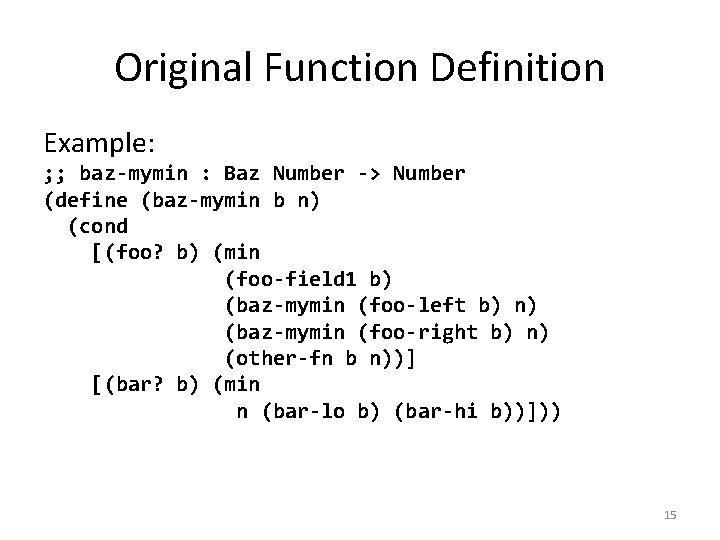Original Function Definition Example: ; ; baz-mymin : Baz Number -> Number (define (baz-mymin b n) (cond [(foo? b) (min (foo-field 1 b) (baz-mymin (foo-left b) n) (baz-mymin (foo-right b) n) (other-fn b n))] [(bar? b) (min n (bar-lo b) (bar-hi b))])) 152. Pull Out the Parts Example: ; ; baz-mymin : Baz Number -> Number (define (baz-mymin b n) (cond For a foo: (min (foo-field 1 b) (baz-mymin (foo-left b) n) (baz-mymin (foo-right b) n) (other-fn b n)) For a bar: (min n (bar-lo b) (bar-hi b)) 163. Change Selectors to field references Example: ; ; baz-mymin : Baz Number -> Number (define (baz-mymin b n) (cond For a foo: (min (foo-left b) is replaced by the field 1 field left. (baz-mymin left n) (baz-mymin right n) A b that appears by itself refers to the whole struct, so it is (other-fn this n)) replaced by this. For a bar: (min n lo hi) 17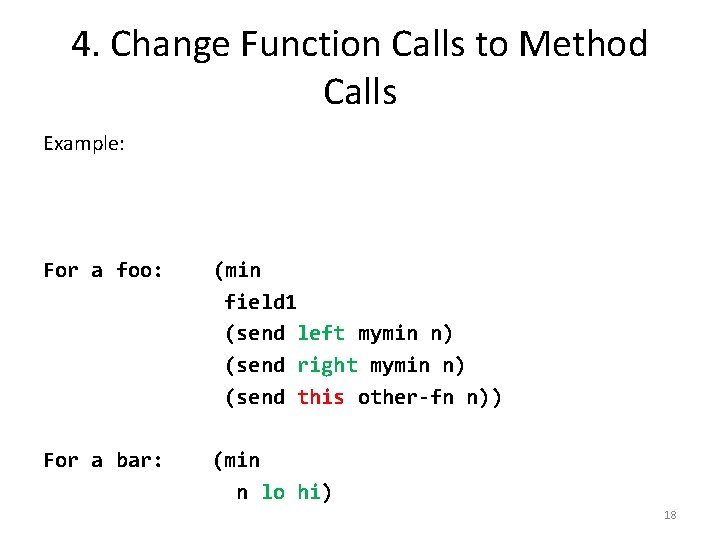4. Change Function Calls to Method Calls Example: ; ; baz-mymin : Baz Number -> Number (define (baz-mymin b n) (cond For a foo: (min field 1 (send left mymin n) (send right mymin n) (send this other-fn n)) For a bar: (min n lo hi) 18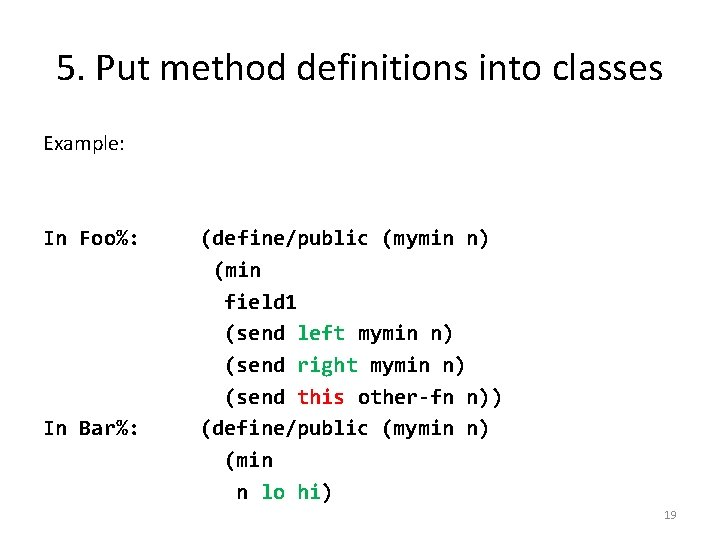5. Put method definitions into classes Example: ; ; baz-mymin : Baz Number -> Number (define (b n) In Foo%: (define/public (mymin n) (min field 1 (send left mymin n) (send right mymin n) (send this other-fn n)) In Bar%: (define/public (mymin n) (min n lo hi) 19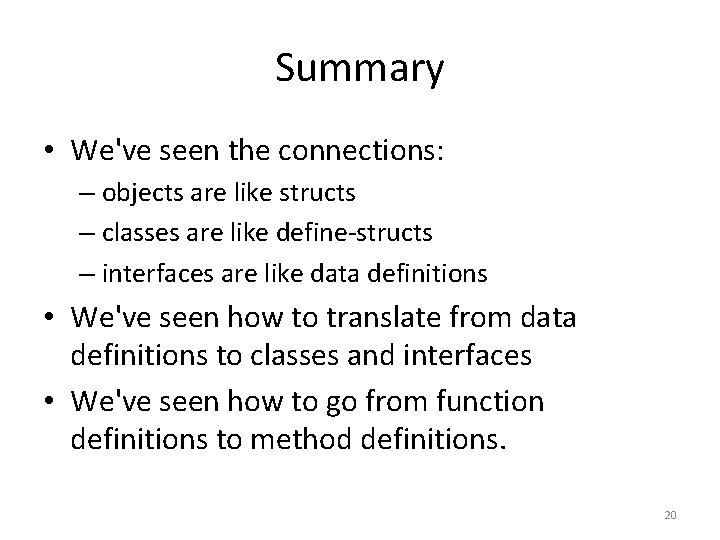Summary • We've seen the connections: – objects are like structs – classes are like define-structs – interfaces are like data definitions • We've seen how to translate from data definitions to classes and interfaces • We've seen how to go from function definitions to method definitions. 20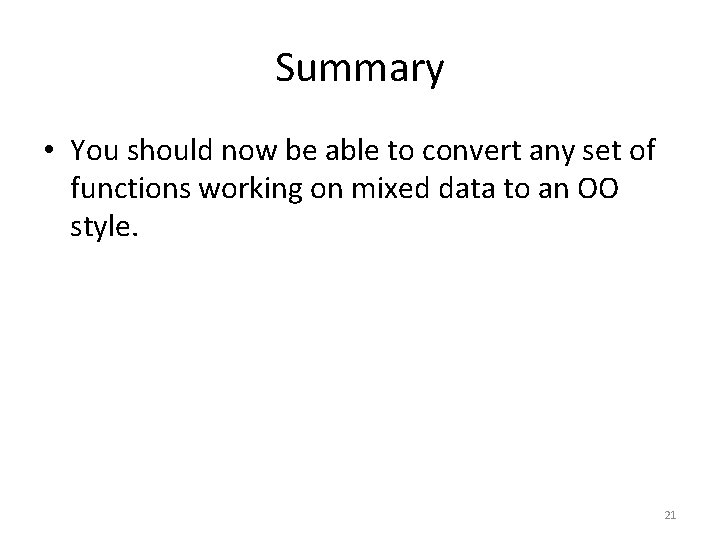Summary • You should now be able to convert any set of functions working on mixed data to an OO style. 21